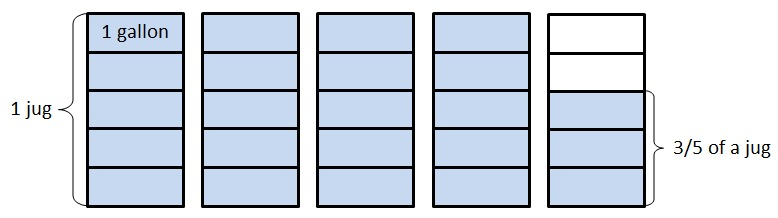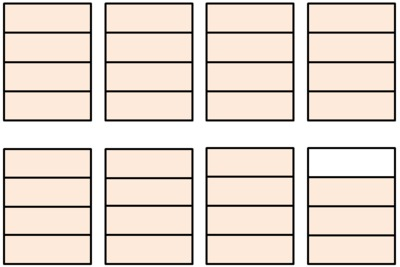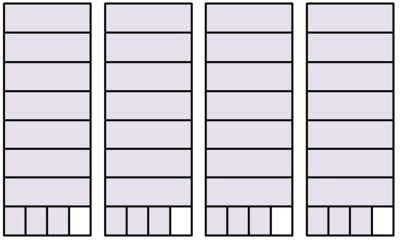# What is 23 ÷ 5?

Alignments to Content Standards: 5.NF.B.3 5.NBT.B.7

1. Jessa has 23 one-dollar bills that she wants to divide equally between her 5 children.
1. How much money will each receive? How much money will Jessa have left over?
2. Jessa exchanged the remaining one-dollar bills for dimes. If she divides the money equally between her 5 children, how much money will each child get?
2. A website has games available to purchase for \$5 each. If Lita has \$23, how many games can she purchase? Explain.
3. A jug holds 5 gallons of water. How many jugs can Mark fill with 23 gallons of water? Explain.
4. A class of 23 children will take a field trip. Each car can take 5 children. How many cars are needed to take all the children on the field trip? Explain.
5. Write a division problem for 31 ÷ 4 where the answer is a mixed number. Show how to solve your problem.

## IM Commentary

When a division problem involving whole numbers does not result in a whole number quotient, it is important for students to be able to decide whether the context requires the result to be reported as a whole number with remainder (as with Part (b)) or a mixed number/decimal (as with Part (c)). Part (a) presents two variations on a context that require these two different responses to highlight the distinction between them. The second variation intentionally exchanges dollars for dimes because the division can be demonstrated very nicely with a chip (or money) model, and it fits precisely with the steps of long division of decimal numbers, helping to lay the foundation for that algorithm (which students are not expected to use until 6th grade; see 6.NS.3). In part (d), students are asked a question that requires division but where the quotient must be rounded up to the next whole number. This task bridges between work students started in fourth grade (see 4.OA.3) and the fifth grade expectations described in 5.NF.3.

The Standards for Mathematical Practice focus on the nature of the learning experiences by attending to the thinking processes and habits of mind that students need to develop in order to attain a deep and flexible understanding of mathematics. Certain tasks lend themselves to the demonstration of specific practices by students. The practices that are observable during exploration of a task depend on how instruction unfolds in the classroom. While it is possible that tasks may be connected to several practices, only one practice connection will be discussed in depth. Possible secondary practice connections may be discussed but not in the same degree of detail.

This particular task helps illustrate Mathematical Practice Standard 1, Make sense of problems and persevere in solving them.  Problem solving is based upon students engaging in tasks in which a solution pathway is not known in advance.  As fifth graders approach these problems, they will analyze them to make sense of what each is asking and decide on the best solution pathways. Students will notice that even though the same numbers are used in problems (a), (b), (c), and (d), the contexts are very different, and it might not be obvious when reading the problem that division is necessary in each context. As students look for similarities/differences between the problems, they observe that problems (b), (c), and (d) ask the question “how many groups?” while problem (a) asks “how many in each group?” These observations will determine their entry points into each problem. Students may also conclude that how the remainder is reported is dependent upon the particular context of each problem. For instance, in problem (b) a remainder is reported but in problem (d) only a whole number quotient is required. Answering to a required degree of precision also supports MP.6, Attend to precision.

## Solution

1. This question asks for the number of dollars each child gets, so is a “how many in each group?” division problem. Additionally, the way the problem is worded, only a whole number of dollars can be in each group. Thus, the extra dollar bills should be reported as a remainder.

Jessa is only giving away whole dollar bills, so each child will receive \$4 and Jessa will have \$3 left.

2. This question asks for the number of dollars each child gets, so is a “how many in each group?” division problem. However, the way the problem is worded, a fraction of a dollar is allowed. Because it is a money context, it makes more sense to report the answer as a decimal rather than a mixed number. \begin{align} 23\div 5 =& 4\frac35 \\ =& 4 \frac{6}{10}\\ =& 4.6 \end{align} So each child will get \$4.60. If students need some additional help in understanding this answer, a picture might look like this:1. This question asks for the number of games Lita can purchase, so is a “how many groups?” division problem. Lita can only purchase a whole number of games, so the extra money should be reported as a remainder. Note that in this case the remainder has a different unit than the quotient, in contrast to the first two problems where the units are the same in both cases. Lita can purchase 4 games and she will have \$3 left over.

2. This question asks for the number of jugs Mark can fill, so is a “how many groups?” division problem. There are two possible answers to this question depending on whether one wants to consider filling a fraction of a jug or if one only wants to consider jugs that are filled all the way to the top. Note that the interpretation of the numbers is very different if the 3 is left as remainder (where the units for the 4 and 3 are different) or if the answer is written as a mixed number (where the 4 and the 3/5 have the same unit).Mark could fill 4 jugs to the top and have 3 gallons of water left over, or he could fill 4 3/5 jugs.

3. This question asks for the number of cars needed for the field trip, so is a “how many groups?” division problem. The difference between this problem and the ones above is that we can't have students left out of the field trip, so we don't want to report the quotient as a whole number with remainder (4 cars are filled and 3 children are left at school) and we can't have a fraction of a car, so we don't want to report the quotient as a mixed number (they need 4 3/5 cars).

Five cars are needed to take all the students on the field trip.

4. There are many possibilities, but they will generally fall into one of two categories: "how many groups?" or "how many in each group?" Here is how a "how many groups?" picture might look:For example, if you have 31 pounds of flour and a bag holds 4 pounds, how many bags can you fill?

Here is how a "how many in each group?" picture might look:For example, if you have 31 pounds of flour and you want to divide it equally into 4 sacks, how many pounds of flour will be in each sack?

In either case, students who draw pictures to represent their solution should label them carefully so it is clear how the picture should be interpreted in the context (see the picture for Part (c)).